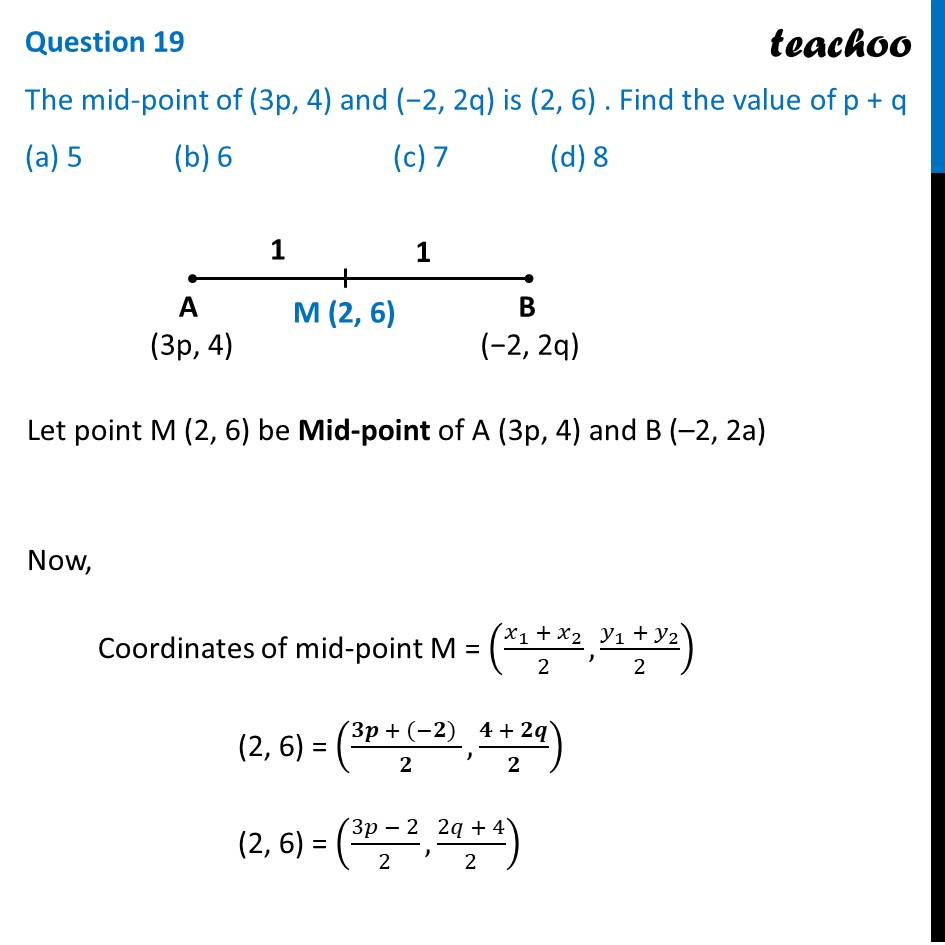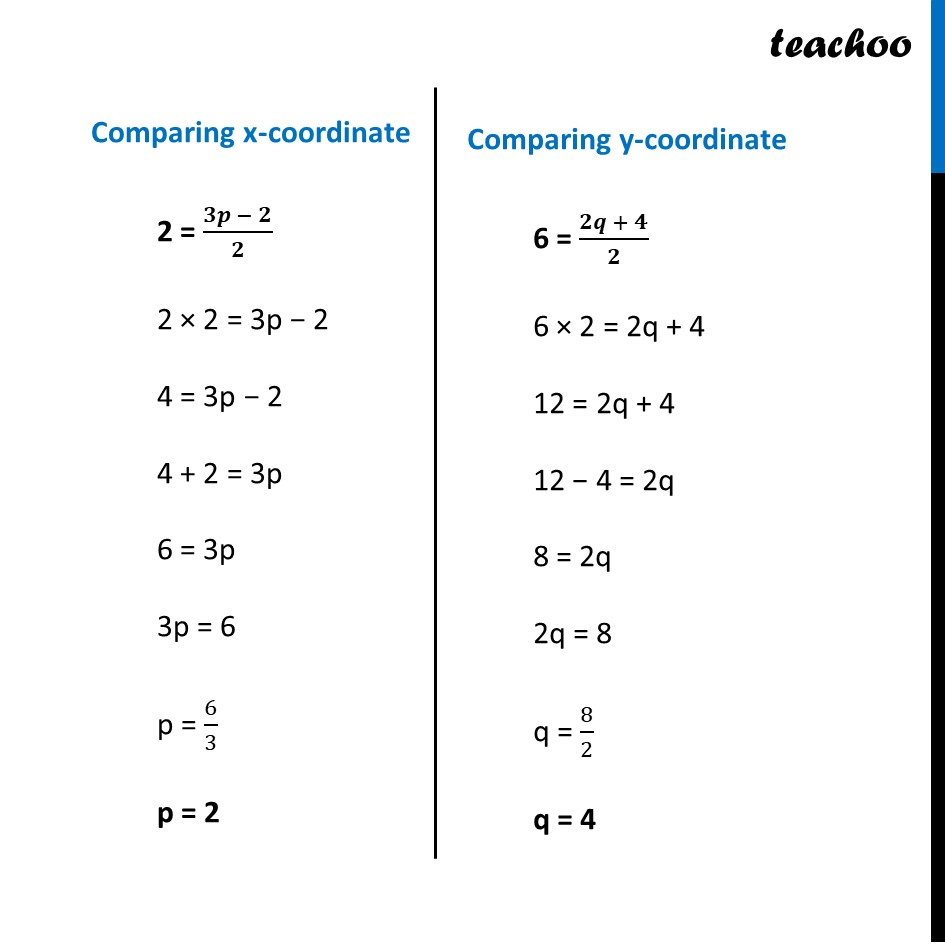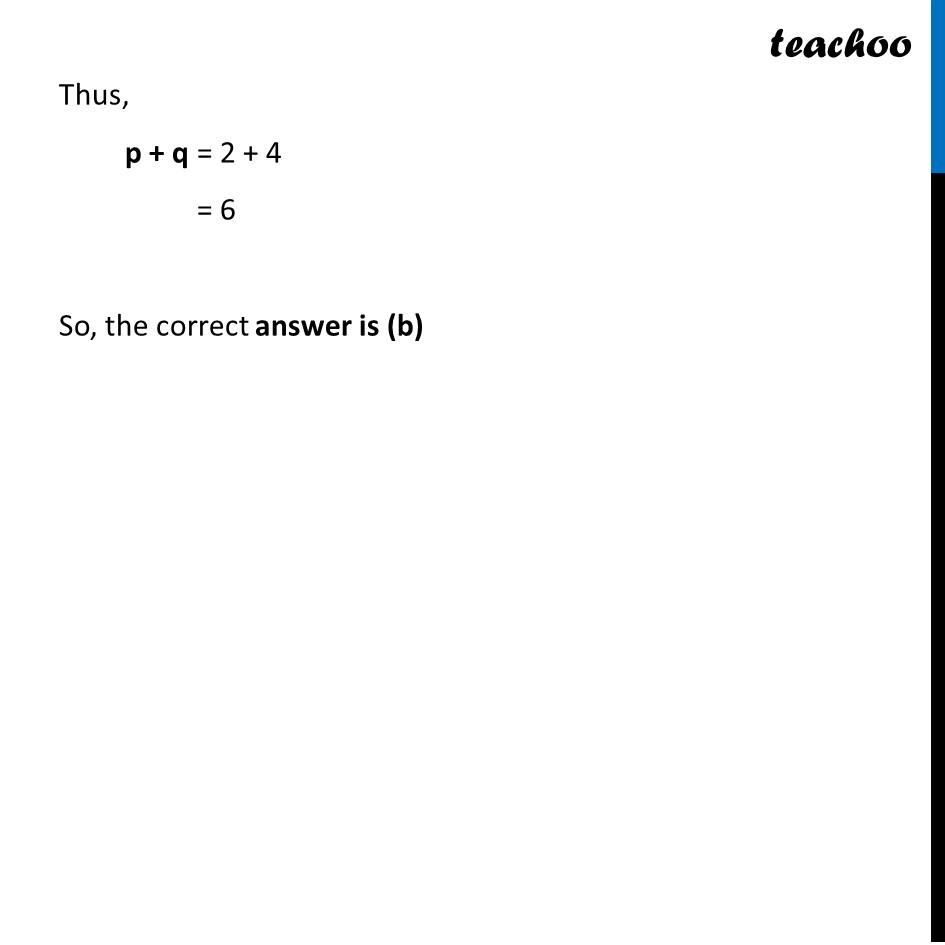CBSE Class 10 Sample Paper for 2022 Boards - Maths Basic [MCQ]

Class 10
Solutions of Sample Papers for Class 10 Boards

## (a) 5  (b) 6        (c) 7        (d) 8Learn in your speed, with individual attention - Teachoo Maths 1-on-1 Class

### Transcript

Question 19 The mid-point of (3p, 4) and (−2, 2q) is (2, 6) . Find the value of p + q (a) 5 (b) 6 (c) 7 (d) 8 Let point M (2, 6) be Mid-point of A (3p, 4) and B (–2, 2a) Now, Coordinates of mid-point M = ((𝑥_1 + 𝑥_2)/2,(𝑦_1 + 𝑦_2)/2) (2, 6) = ((𝟑𝒑 + (−𝟐) )/𝟐,(𝟒 + 𝟐𝒒)/𝟐) (2, 6) = ((3𝑝 − 2)/2,(2𝑞 + 4)/2) Comparing x-coordinate 2 = (𝟑𝒑 − 𝟐)/𝟐 2 × 2 = 3p − 2 4 = 3p − 2 4 + 2 = 3p 6 = 3p 3p = 6 p = 6/3 p = 2 Comparing y-coordinate 6 = (𝟐𝒒 + 𝟒)/𝟐 6 × 2 = 2q + 4 12 = 2q + 4 12 − 4 = 2q 8 = 2q 2q = 8 q = 8/2 q = 4 Thus, p + q = 2 + 4 = 6 So, the correct answer is (b)